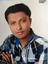Trending ▼   ResFinder# CBSE Pre Board Class 12 2017 : Computer Science (Maharashtra Higher Sec. School, Jabalpur) Test 1

6 pages, 54 questions, 0 questions with responses, 0 total responses,20rajan53 Maharashtra Higher Sec. School, Jabalpur
+Fave Message
 Home > rajan53 >   F Also featured on: School Page cbse12_pre_boardsFormatting page ...

Sub.- Computer Science [C++] Class- XII MM. -70 Time- 3 Hrs. Q1. What is OOP s and their concepts? 1 Q2. What are static functions? 1 Q3. What is local and global class? 1 Q4. Write the names of the header files to which the following belong 1. Getline() 2. Strcmp() 1 Q5. What is a spam mail? 1 Q6. Which C++ header file(s) will be essentially required to be included to run /execute the following C++ code: 1 void main() { char Msg[ ]="Sunset Gardens"; for (int I=5;I<strlen(Msg);I++) puts(Msg); } Q7. What is Recursion? Give example also. 2 Q8. In the following program, if the value of N given by the user is 15, what maximum and minimum values the program could possibly display? 2 #include <iostream.h> #include <stdlib.h> void main() { int N,Guessme; randomize(); cin>>N; Guessme=random(N)+10; cout<< \n <<Guessme; } Q9. What is the output of following program? 3 1 int x=1o,y; 2. int x=10,y; 3. cout << setw(5) << 77 <<endl; y=x + x++; y=++x + x++ + x; cout << setw(5) << 100 <<endl; cout<<y; cout<<y; cout << setw(5) << 12312<<endl; Q10. What do you understand by Degree and Cardinality of a table? 2 Q11. Name the function/method required to : 2 (i) check if a string contains only uppercase letters (ii) gives the total length of the string. Q12. What do you understand by Polymorphism? Give a suitable example of the same. 2 Q13. Write a function in C++ to find sum of rows from a two dimensional array. 2 Q14. Reads the given code carefully and rewrite it after removing all syntactical errors with each correction underlined. Note: Assume all required header files are already being included in the program. 2 #Define float Max=70.0; Void main() { int Speed char Stop= N ; cin>>Speed; if Speed>Max Stop= Y ; cout<<Stop<<end; } Q15. Answer the questions (i) and (ii) after going through the following class: 2 class Seminar { int Time; public: Seminar() //Function 1Formatting page ...Formatting page ...Formatting page ...Formatting page ...Formatting page ...© 2010 - 2021 ResPaper. Terms of ServiceContact Us Advertise with us

ICSE Q&A - Ask and Answerrajan53 chat